# Solutions to Review 1Question 1
(i)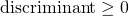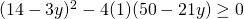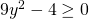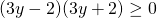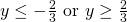(ii)
Using long division, we find that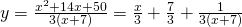So the asymptotes areandQuestion 2
(i)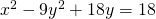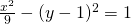This is a hyperbola with centre, asymptotes are, and verticesand.is a graph with asymptotesand.

Use GC to plot.

(ii)—(1)—(2)

Subst (2) to (1),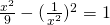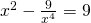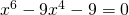(iii)
From graph, we observe two intersections. Thus, two roots.

Question 3
(ai)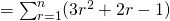(aii)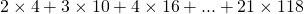(bi)Let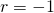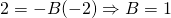Let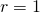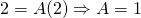(bii)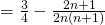(biii)
As,and, the sum of series tends to, a constant. Thus, series is convergent.

(biv)Replaceby. Then we haveNot readable? Change text.